Importance: High ✭✭✭
 Subject: Graph Theory » Infinite Graphs
 Keywords: binary tree infinite graph normal spanning tree set theory
 Posted by: mdevos on: June 26th, 2008

Call a graph an-graph if it has a bipartition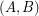so that every vertex inhas degreeand every vertex inhas degree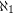.

Problem   Characterize the-graphs.

The motivation for this problem comes from a lovely paper of Diestel and Leader [DL] where they prove that an infinite graph has a normal spanning tree (the natural infinite analogue of a depth-first search tree) if and only if it has no minor isomorphic to either an-graph or an Aronszajn tree. (An earlier conjecture of Halin asserted that only the first of these excluded minors was needed.) So,-graphs appear as a forbidden minor obstruction to the existence of a kind of depth-first search tree for infinite graphs.

The obvious example of an-graph is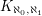, but there are other natural families of such graphs. For instance, letbe an infinite binary tree with root, and letbe the set of all rays (one way infinite paths) with endpoint. Now, form a bipartite graph with vertex bipartitionand adjacency given by the rule that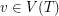adjacent to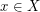if and only iflies on the ray(in). Any-graph which is isomorphic to a subgraph of this graph is said to be of binary type.

Say that a-graph is divisible if there exist disjoint subsets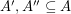and disjoint subsetsso that the graphs induced by bothandare-graphs. It is not difficult to show that every binary type graph is divisible. Curiously, the existence of non-divisible-graphs depends on the Continuum Hypothesis (see [DL]).

Although it is not clear wether or not there is a nice characterization of-graphs, it would certainly be interesting to find more natural families of these graphs. The following rather more concrete question is posed by Diestel and Leader who suspect the answer is 'no'.

Problem   Does every-graph have an-graph as a minor which is either indivisible or of binary type?

## Bibliography

[DL] R. Diestel and I. Leader, Normal spanning trees, Aronszajn trees and excluded minors, J. London Math. Soc. 63 (2001), 16-32;

* indicates original appearance(s) of problem.